# Nodes Panel

Use the Nodes panel to create nodes using a wide variety of methods.

Location: Geom page

##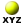XYZ Subpanel

Use the XYZ subpanel to create nodes by specifying (x,y,z) coordinates.
There are several methods for entering the coordinate values.
• Manually enter the coordinate values in the x, y, and z fields.
• Use the x, y, z selectors to populate the x, y, and z values by selecting nodes in the modeling window. Upon selecting a node, the respective x, y, or z coordinate value is automatically displayed in the corresponding field. The values can be edited as desired.
• Use the as node selector to populate the coordinate and system values by selecting a node in the modeling window. Upon selecting a node, the respective x, y and z coordinate values and the reference system value from the selected node is automatically displayed in the corresponding field. The values can be edited as desired.

##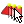On Geometry Subpanel

By default, nodes are created relative to the global system, however a local coordinate system can be specified using the system selector. This system can be rectangular, cylindrical or spherical. When a node is created, it is also assigned to the specified reference system. The node is then updated to reflect any changes that are made to that system (for example translate, rotate).

Use the On Geometry subpanel to create nodes at graphically selected locations on points, lines, surfaces and planes. Upon selecting the type of geometry you wish to create nodes on, you can click any location on such geometry in the modeling window to place a node. In the case of nodes on a plane, you must first create the plane, which is temporary and invisible, but allows you to place nodes outside of existing geometry.

##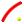Arc Center Subpanel

Use the Arc Center subpanel to create a node at the center of the arc that best approximates the input set of nodes, points, or lines.

For nodes and points input, a minimum of three must be selected as shown in Figure 5.
For lines input, the selection must not result in a straight line. An existing arc is an ideal input as shown in Figure 6. However, almost any set of lines will also work, even if they are not connected, as long as they do not form a straight line as shown in Figure 7.

An optional tolerance is available to verify whether the calculated best-fit circle is sufficient. If ignored, the best-fit circle is always calculated; otherwise, an error is returned if the input entities are not all on the circle within the specified tolerance.

##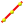Extract Parametric Subpanel

Use the Extract Parametric subpanel to create nodes at parametric locations on lines and surfaces.

For lines:
• 0.0 <= u lower bound <= u upper bound <= 1.0.
• If the lower and upper bounds are the same, only one node is created.
• If the number of u nodes is specified as 1, only the lower bound is used.
• The line parameterization type can be specified as either arc length or internal. Internal parameterizations depend on how the line was originally created, while arc length simply distributes the nodes uniformly.
For surfaces:
• 0.0 <= u lower bound <= u upper bound <= 1.0.
• 0.0 <= v lower bound <= v upper bound <= 1.0.
• The number of u and v nodes must be >= 1.
• The total number of nodes created equals (number of u nodes * number of v nodes).
• The surface parametric area is scaled to the visible surface area. Nodes will be created inside the visible surface area.

##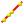Extract on Line Subpanel

Use the Extract on Line subpanel to create evenly spaced or biased nodes on a selection of lines.

Figure 10, Figure 11, and Figure 12 use the same input line, 7 nodes, and a bias intensity of 5.0.

##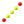Interpolate Nodes Subpanel

Use the Interpolate Nodes subpanel to create evenly spaced or biased nodes by interpolating between existing nodes in space.

The input nodes are specified as a node list, with each node considered as a pair with the next node in the list. The specified number of nodes are created on a straight line between each node pair.

Figure 13 shows the effect of adding 5 nodes between the end nodes of a line using different types of bias, with bias intensity 5.0.
Nodes are created in 3D space, and not merely on geometry as shown in Figure 14.

To interpolate nodes along a curved line, use the Interpolate on Line subpanel instead.

##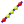Interpolate on Line Subpanel

Use the Interpolate on Line subpanel to create evenly spaced or biased nodes by interpolating between existing nodes on a line.

The input nodes are specified as a node list, with each node considered as a pair with the next node in the list. The nodes are created on the input line between each node pair.

The input nodes are not required to lie on the selected line, as all input nodes are first projected onto the line.

##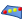Interpolate on Surface Subpanel

Use the Interpolate on Surface subpanel to create evenly spaced or biased nodes by interpolating between existing nodes on a surface.

The input nodes are specified as a node list, with each node considered as a pair with the next node in the list. The nodes are created on the input surface between each node pair.

The nodes are not required to lie on the selected surface, as all input nodes are first projected onto the surface.

##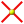Intersect Subpanel

Use the Intersect subpanel to create nodes at the intersection of geometric entities, such as lines/lines, lines/surfaces, lines/solids, lines/planes, vector/lines, vector/surfaces, vector/solids and vector/plane.

For surface, solid, and plane intersections, if the input line or vector lies on the surface/solid/plane the result is undefined and unpredictable.

For vectors, the magnitude and direction of the vector do not have any effect on the result. The vector extends through the entire model space.